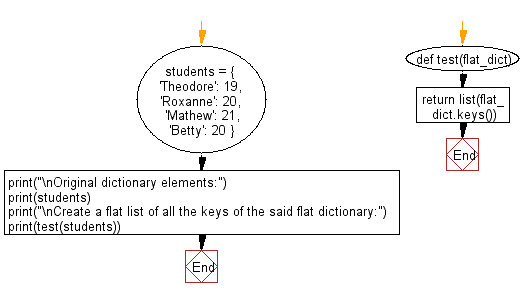﻿ Python: Create a flat list of all the keys in a flat dictionary - w3resource# Python: Create a flat list of all the keys in a flat dictionary

## Python dictionary: Exercise-78 with Solution

Write a Python program to create a flat list of all the keys in a flat dictionary.

• Use dict.keys() to return the keys in the given dictionary.
• Return a list() of the previous result.

Sample Solution:

Python Code:

``````def test(flat_dict):
return list(flat_dict.keys())
students = {
'Theodore': 19,
'Roxanne': 20,
'Mathew': 21,
'Betty': 20
}

print("\nOriginal dictionary elements:")
print(students)
print("\nCreate a flat list of all the keys of the said flat dictionary:")
print(test(students))
```
```

Sample Output:

```Original dictionary elements:
{'Theodore': 19, 'Roxanne': 20, 'Mathew': 21, 'Betty': 20}

Create a flat list of all the keys of the said flat dictionary:
['Theodore', 'Roxanne', 'Mathew', 'Betty']
```

Flowchart:## Visualize Python code execution:

The following tool visualize what the computer is doing step-by-step as it executes the said program:

Python Code Editor:

Have another way to solve this solution? Contribute your code (and comments) through Disqus.

What is the difficulty level of this exercise?

Test your Programming skills with w3resource's quiz.

﻿

## Python: Tips of the Day

Decapitalizes the first letter of a string:

Example:

```def tips_decapitalize(s, upper_rest=False):
return s[:1].lower() + (s[1:].upper() if upper_rest else s[1:])
print(tips_decapitalize('PythonTips'))
print(tips_decapitalize('PythonTips', True))
```

Output:

```pythonTips
pYTHONTIPS
```